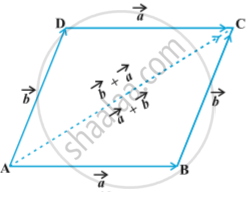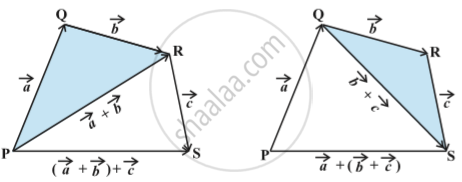#### text

Property:-  For any two vectors vec aand vec b,
vec a + vec b = vec b + vec a         (Commutative property)
Proof: Consider the parallelogram ABCDLet vec (AB) = vec a and vec (BC) = vec b, then using the triangle law, from triangle ABC , we have vec (AC) = vec a + vec b
Now, since the opposite sides of a parallelogram are equal and parallel, from abov fig.
we have , vec (AD) = vec (BC) = vec b and vec (DC) = vec (AB) = vec a
Again using triangle law, from triangle ADC, we have  vec (AC) = vec (AD) + vec (DC) = vec b + vec a
Hence vec a + vec b = vec b + vec a

Property:-  For any three vectors vec a , vec b and vec c
(vec a + vec b) + vec c = vec a + (vec b + vec c)
(Associative property)
Proof: Let the vectors vec a , vec b and vec c be represented by vec (PQ) , vec (QR) and vec (RS), respectively , as shown in following fig.Then vec a + vec b = vec (PQ) + vec (QR) = vec (PR)

and  vec b + vec c = vec (QR) + vec (RS) = vec (QS)

So, (vec a + vec b) + vec c = vec (PR) + vec (RS) = vec (PS)

and vec a + (vec b+vec c) = vec (PQ) + vec (QS) = vec (PS)

Hence (vec a + vec b) + vec c = vec a + (vec b+vec c)

Remark:
Any vector vec a, we have
vec a + vec 0 =vec 0 + vec a = vec a
Here, the zero vector vec 0  is called the additive identity for the vector addition.

If you would like to contribute notes or other learning material, please submit them using the button below.

### Shaalaa.com

Vector Algebra part 7 (Properties of Vector addition) [00:03:37]
S
0%